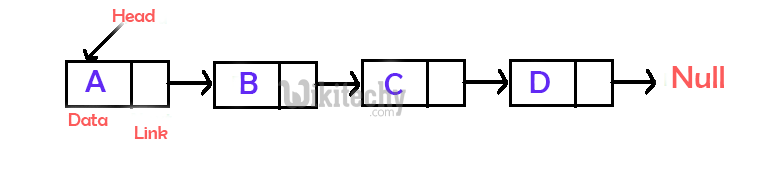# Insertion Sort for Singly Linked List

Insertion Sort for Singly Linked List - Searching and sorting - We have discussed Insertion Sort for arrays.In this article linked list is also discussed.

Singly Linked List is a collection of ordered set of elements. A Node in singly linked list has two parts – data part and link part. Data part of the node contains the actual information which is represented as node. Link part of the node contains address of next node linked to it.

It can be traversed in only one direction because the node stores only next pointer. So, it can’t reverse linked list.### Algorithm for Insertion Sort for Singly Linked List :

•  Create an empty sorted (or result) list
•  Traverse the given list, do following for every node.
•  Insert current node in sorted way in sorted or result list.

### Insertion Sort for Singly Linked List:

In C language, program is given below:

c
``````/* C program for insertion sort on a linked list */
#include<stdio.h>
#include<stdlib.h>

struct node
{
int data;
struct node* next;
};

// Function to insert a given node in a sorted linked list
void sortedInsert(struct node**, struct node*);

// function to sort a singly linked list using insertion sort
{
struct node *sorted = NULL;

// Traverse the given linked list and insert every
// node to sorted
while (current != NULL)
{
// Store next for next iteration
struct node *next = current->next;

// insert current in sorted linked list
sortedInsert(&sorted, current);

// Update current
current = next;
}

}

/* function to insert a new_node in a list. Note that this
function expects a pointer to head_ref as this can modify the
void sortedInsert(struct node** head_ref, struct node* new_node)
{
struct node* current;
/* Special case for the head end */
{
}
else
{
/* Locate the node before the point of insertion */
while (current->next!=NULL &&
current->next->data < new_node->data)
{
current = current->next;
}
new_node->next = current->next;
current->next = new_node;
}
}

/* BELOW FUNCTIONS ARE JUST UTILITY TO TEST sortedInsert */

/* Function to print linked list */
{
while(temp != NULL)
{
printf("%d  ", temp->data);
temp = temp->next;
}
}

/* A utility function to insert a node at the beginning of linked list */
void push(struct node** head_ref, int new_data)
{
/* allocate node */
struct node* new_node = new node;

/* put in the data  */
new_node->data  = new_data;

/* link the old list off the new node */

/* move the head to point to the new node */
}

// Driver program to test above functions
int main()
{
struct node *a = NULL;
push(&a, 5);
push(&a, 20);
push(&a, 4);
push(&a, 3);
push(&a, 30);

printList(a);

insertionSort(&a);

printList(a);

return 0;
}``````

### OUTPUT:

```Linked List before sorting
30  3  4  20  5